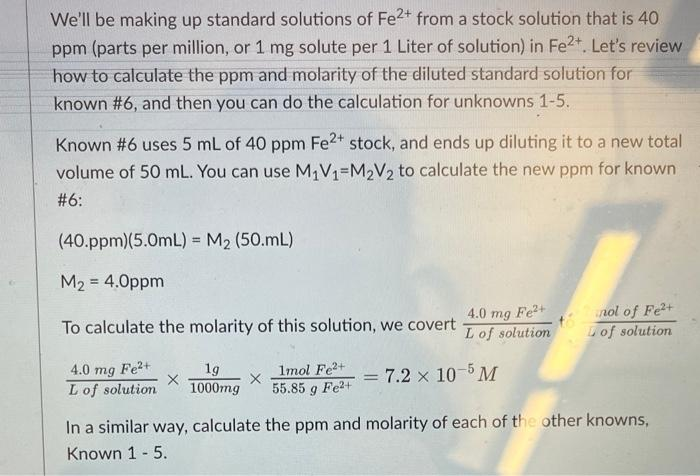Home / Expert Answers / Chemistry / we-39-ll-be-making-up-standard-solutions-of-mathrm-fe-2-from-a-stock-solution-that-is-40-pp-pa920

# (Solved): We'll be making up standard solutions of $$\mathrm{Fe}^{2+}$$ from a stock solution that is 40 pp ...We'll be making up standard solutions of $$\mathrm{Fe}^{2+}$$ from a stock solution that is 40 ppm (parts per million, or 1 mg solute per 1 Liter of solution) in $$\mathrm{Fe}^{2+}$$. Let's review how to calculate the ppm and molarity of the diluted standard solution for known #6, and then you can do the calculation for unknowns 1-5. Known #6 uses $$5 \mathrm{~mL}$$ of $$40 \mathrm{ppm} \mathrm{Fe}^{2+}$$ stock, and ends up diluting it to a new total volume of $$50 \mathrm{~mL}$$. You can use $$M_{1} V_{1}=M_{2} V_{2}$$ to calculate the new ppm for known #6: $$(40 . \mathrm{ppm})(5.0 \mathrm{~mL})=\mathrm{M}_{2}(50 . \mathrm{mL})$$ $$M_{2}=4.0 p p m$$ To calculate the molarity of this solution, we covert $$\frac{4.0 \mathrm{mg} \mathrm{Fe}}{\mathrm{L} \text { of solution }}+\frac{\text { nol of } \mathrm{Fe}^{2+}}{\sqrt{\text { of solution }}}$$ In a similar way, calculate the ppm and molarity of each of the other knowns, Known $$1-5$$

We have an Answer from Expert